Procedures for Weighing an Aircraft | Aircraft Systems

# Procedures for Weighing an Aircraft

## General Concepts

The most important reason for weighing an aircraft is to find out its empty weight (basic empty weight) and to find out where it balances in the empty weight condition. When an aircraft is to be flown, the pilot in command must know what the loaded weight of the aircraft is and where its loaded CG is. For the loaded weight and CG to be calculated, the pilot or dispatcher handling the flight must first know the empty weight and EWCG.

In this site it was identified that the CG for an object is the point about which the nose heavy and tail heavy moments are equal. One method that could be used to find this point would involve lifting an object off the ground twice, first suspending it from a point near the front, and on the second lift suspending it from a point near the back. With each lift, a perpendicular line (90 degrees) would be drawn from the suspension point to the ground. The two perpendicular lines would intersect somewhere in the object, and the point of intersection would be the CG. This concept is shown in Figure 1, where an airplane is suspended from two different points.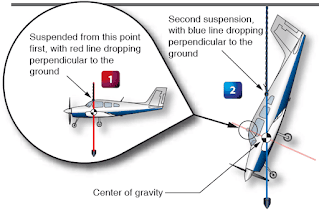Figure 1. Center of gravity determined by two suspension points

The perpendicular line from the first suspension point is shown in red, and the new suspension point line is shown as a blue plumb bob. Where the red and blue lines intersect is the CG. If an airplane were suspended from two points, one at the nose and one at the tail, the perpendicular drop lines would intersect at the CG. Suspending an airplane from the ceiling by two hooks, however, is clearly not realistic. Even if it could be done, determining where in the airplane the lines intersect would be difficult.

A more realistic way to find the CG for an object, especially an airplane, is to place it on a minimum of two scales and calculate the moment value for each scale reading. In Figure 2, there is a plank that is 200" long, with the left end being the datum (zero arm), and 6 weights placed at various locations along the length of the plank. The purpose of Figure 2 is to show how the CG can be calculated when the arms and weights for an object are known.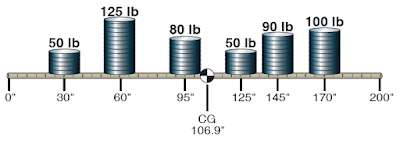Figure 2. Center of gravity for weights on a plank with datum at one end

To calculate the CG for the object in Figure 2, the moments for all the weights need to be calculated and then summed, and the weights need to be summed. In the four-column table in Figure 3, the item, weight, and arm are listed in the first three columns, with the information coming from Figure 2. The moment value in the fourth column is the product of the weight and arm. The weight and moment columns are summed, with the CG being equal to the total moment divided by the total weight. The arm column is not summed. The number appearing at the bottom of that column is the CG. The calculation is shown in Figure 3.Figure 3. Center of gravity calculation for weights on a plank with datum at one end

For the calculation in Figure 3, the total moment is 52,900 in-lb, and the total weight is 495 lb. The CG is calculated as follows:

CG = Total Moment ÷ Total Weight
= 52,900 in-lb ÷ 495 lb
= 106.9" (106.87 rounded to tenths)

An interesting characteristic exists for the problem in Figure 2 and the table showing the CG calculation. If the datum (zero arm) for the object was in the middle of the 200" long plank, with 100" of negative arm to the left and 100" of positive arm to the right, the solution would show the CG to be in the same location. The arm for the CG would not be the same number, but its physical location would be the same. Figures 4 and 5 show the new calculation.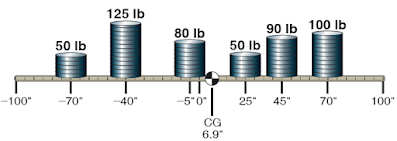Figure 4. Center of gravity for weights on a plank with datum in the middleFigure 5. Center of gravity calculation for weights on a plank with datum in the middle

CG = Total Moment ÷ Total Weight
= 3,400 in-lb ÷ 495 lb
= 6.9" (6.87 rounded to tenths)

In Figure 4, the CG is 6.9" to the right of the plank’s center. Even though the arm is not the same number, in Figure 2 the CG is also 6.9" to the right of center (CG location of 106.9 with the center being 100). Because both problems are the same in these two figures, except for the datum location, the CG must be the same.

The definition for CG states that it is the point about which all the moments are equal. We can prove that the CG for the object in Figure 4 is correct by showing that the total moments on either side of this point are equal. Using 6.87 as the CG location for slightly greater accuracy, instead of the rounded off 6.9 number, the moments to the left of the CG are shown in Figure 6. The moments to the right of the CG, shown in Figure 4, would be as indicated in Figure 7. Disregarding the slightly different decimal value, the moment in both previous calculations is 10,651 in-lb. Showing that the moments are equal is a good way of proving that the CG has been properly calculated.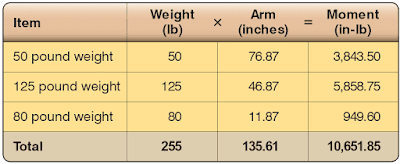Figure 6. Moments to the left of the center of gravity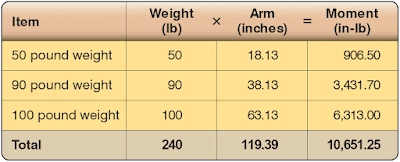Figure 7. Moments to the right of the center of gravity

## Weight and Balance Data

Before an aircraft can be properly weighed and its EWCG computed, certain information must be known. This information is furnished by the FAA to anyone for every certificated aircraft in the TCDS or Aircraft Specifications. When the design of an aircraft is approved by the FAA, an Approved Type Certificate and TCDS are issued. The TCDS includes all the pertinent specifications for the aircraft, and at each annual or 100-hour inspection, it is the responsibility of the inspecting mechanic or repairman to ensure that the aircraft adheres to them.

## Manufacturer-Furnished Information

When an aircraft is initially certificated, its empty weight and EWCG are determined and recorded in the weight and balance record, such as the one in Figure 8. An equipment list is furnished with the aircraft that specifies all the required equipment and all equipment approved for installation in the aircraft. The weight and arm of each item is included on the list, and all equipment installed when the aircraft left the factory is checked. When an aircraft mechanic or repairman adds or removes any item on the equipment list, he or she must change the weight and balance record to indicate the new empty weight and EWCG, and the equipment list is revised to show what is installed.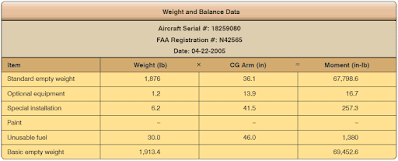Figure 8. Typical weight and balance data for 14 CFR part 23 airplane

Figure 9 is an excerpt from a comprehensive equipment list that includes all the items of equipment approved for this model of aircraft. The Pilot’s Operating Handbook (POH) or Airplane Flight Manual (AFM) for each individual aircraft includes an aircraft specific equipment list of the items from this master list. When any item is added to or removed from the aircraft, its weight and arm are determined in the equipment list and used to update the weight and balance record. The POH/AFM also contains CG moment envelopes and loading graphs.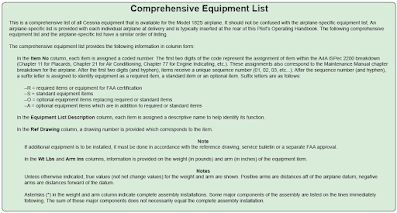Figure 9. Excerpt from a typical comprehensive equipment list

Figures 10 through 12 shows a TCDS for a Piper twin-engine airplane known as the Seneca (PA-34-200). The main headings for the information contained in a TCDS are included, but much of the information contained under these headings has been removed if it did not directly pertain to weight and balance. Information on only one model of Seneca is shown, because to show all the different models would make the document excessively long. The portion of the TCDS that has the most direct application to weight and balance is highlighted in yellow.Figure 10. The Type Certificate Data Sheet (TCDS) shows various information about an aircraft to include weight and balance information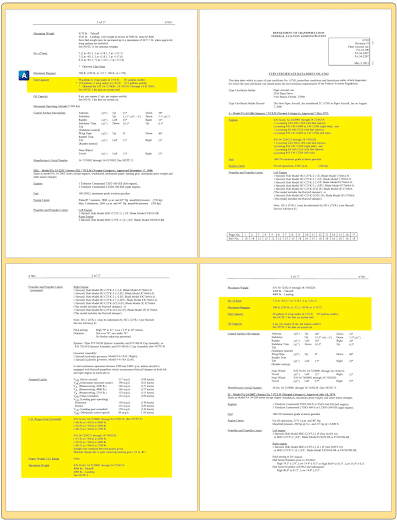Figure 11. Highlights of various specifications of the aircraft found within a TCDS. Note the fuel capacity of 98 gallons and its reference to the datum (A)Figure 12. Highlights of various specifications of the aircraft found within a TCDS

Some of the important weight and balance information found in a TCDS is as follows:
1. Engine
2. CG range
3. Maximum weight
4. Number of seats
5. Baggage capacity
6. Fuel capacity
7. Oil capacity
8. Datum information
9. Leveling means
10. Amount of oil in empty weight
11. Amount of fuel in empty weight

RELATED POSTS